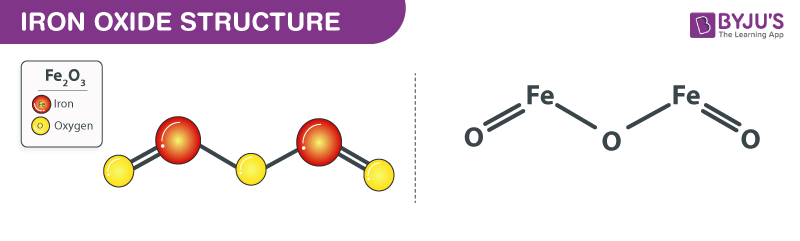# Fe2O3 - Iron(III) oxide

## What is Iron(III) oxide?

Fe2O3 is an inorganic compound with a chemical name Iron(III) oxide. It is also known as Hematite or Red iron oxide. This compound occurs naturally in rocks of all ages. It appears as a Red-brown solid. It is odorless. It has a pH value of 7.

### Production of Iron(III) oxide:

Iron(III) oxide is a product obtained from the oxidation of iron. In laboratories, it is prepared by electrolyzing sodium bicarbonate solution, an inert electrolyte, along with an iron anode.

$4Fe+3O_{2}+2H_{2}O$→$4FeO ( O H)$

The deriving hydrated iron(III) oxide, which is written here as Fe(O)OH, dehydrates at around 200 °C. The reaction is as follows:

$2FeO ( O H)$→$Fe_{2}O_{3}+H_{2}O$

### Properties of Iron(III) oxide – Fe2O3

 Fe2O3 Iron(III) oxide Molecular Weight/ Molar Mass 159.69 g/mol Density 5.242 g/cm³ Boiling Point Decomposes Melting Point 1475°C – 1565°C

### Iron(III) oxide structure – Fe2O3The above image describes the structure of the Iron(III) oxide. Fe2O3 is the chemical formula of Iron(III) oxide which has three oxygen atoms, two iron atoms. The oxidation state of Fe2O3 is +3 and +2. The bond formation between oxygen and iron depends on the difference in electronegativity between these two atoms. Iron(Fe) is metal whereas oxygen(O2) is non-metal. Therefore, such bonds are called an Ionic bond.

Atoms:

 2 iron atoms 3 oxygen atoms Fe O

### Chemical reaction:

1. Carbothermal reduction – Reduction of metal oxides with a reducing agent such as carbon at a temperature range of several hundred degrees Celsius. The reaction is as follows:
$Fe_{2}O_{3}+3CO\rightarrow 2Fe+3CO_{2}$
1. Exothermic thermite reaction with aluminum –

The reaction is as follows:

$2Al+Fe_{2}O_{3}\rightarrow 2Fe+Al_{2}O_{3}$

### Uses of Iron(III) oxide (Fe2O3)

• Iron(III) oxide is used as a feedstock in the production of iron.
• It is used as a pigment. Example: Pigment Brown 6 and Pigment Red 101
• It is used in cosmetics.
• It is used in dental composites.
• It is an important ingredient in calamine lotion.
• It is used to apply the final polish on metallic jewelry.
• It is used in the magnetic disk and magnetic tapes.

Iron(III) oxide health hazards – If inhaled, iron causes irritation in the gastrointestinal tract and lungs.